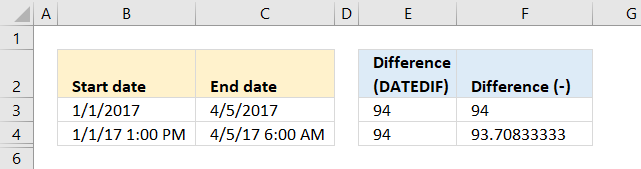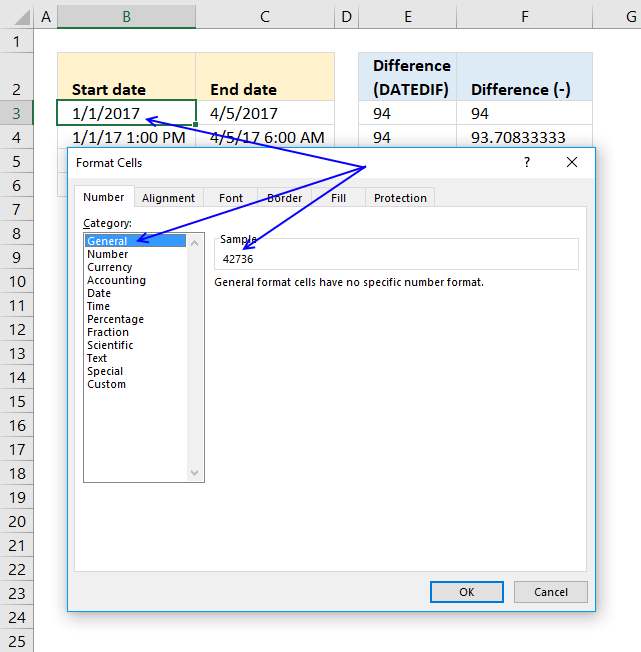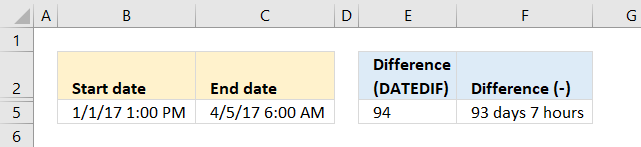Author: Oscar Cronquist Article last updated on March 25, 2018The DATEDIF function in cell E3 allows you to calculate days between two dates.

=DATEDIF(B3,C3,"d")

The function is hidden in the formula bar and the arguments are not shown surprisingly. The first argument is the start date, the second is the end date. The third is the unit.

There seems to be an issue with days between two dates if you use date and time as an argument.

The DATEDIF function should return complete days, however, in this case, it rounds the number up to the nearest whole number. The picture above shows you this issue in cell E4.

=C3-B3

A much easier formula is to simply subtract the earlier date from the later date. Excel dates are actually numbers between 1 and 99999 formatted as dates, this allows you to do mathematical operations to dates.

You can see that yourself by selecting a cell containing a date and then press CTRL + 1. This opens a dialog box where you can see how the cell is formatted.Click on General to show the number. 1/1/2017 is in fact 42736. Number 1 is 1/1/1900.If you use dates and time and want to calculate the number of days and hours between two dates use the following formula:

=INT(C5-B5)& " days "&HOUR(B5-C5-INT(B5-C5))&" hours"

The result is displayed in cell F5 on the picture above.

INT(C5-B5)& " days "

The INT function removes the decimal part from the number returning complete days. The & (ampersand) concatenates the number with the text string " days".

HOUR(B5-C5-INT(B5-C5))&" hours"

The HOUR function returns a number representing the hour. The decimal part of the number is the time, in this case, hours.

To get the decimal part simply subtract the integer part from the number, this is where the INT function comes in.

Lastly, the ampersand & character concatenates the hour number with " hours".

You can get even greater detail by using the MINUTE and SECOND functions as well.